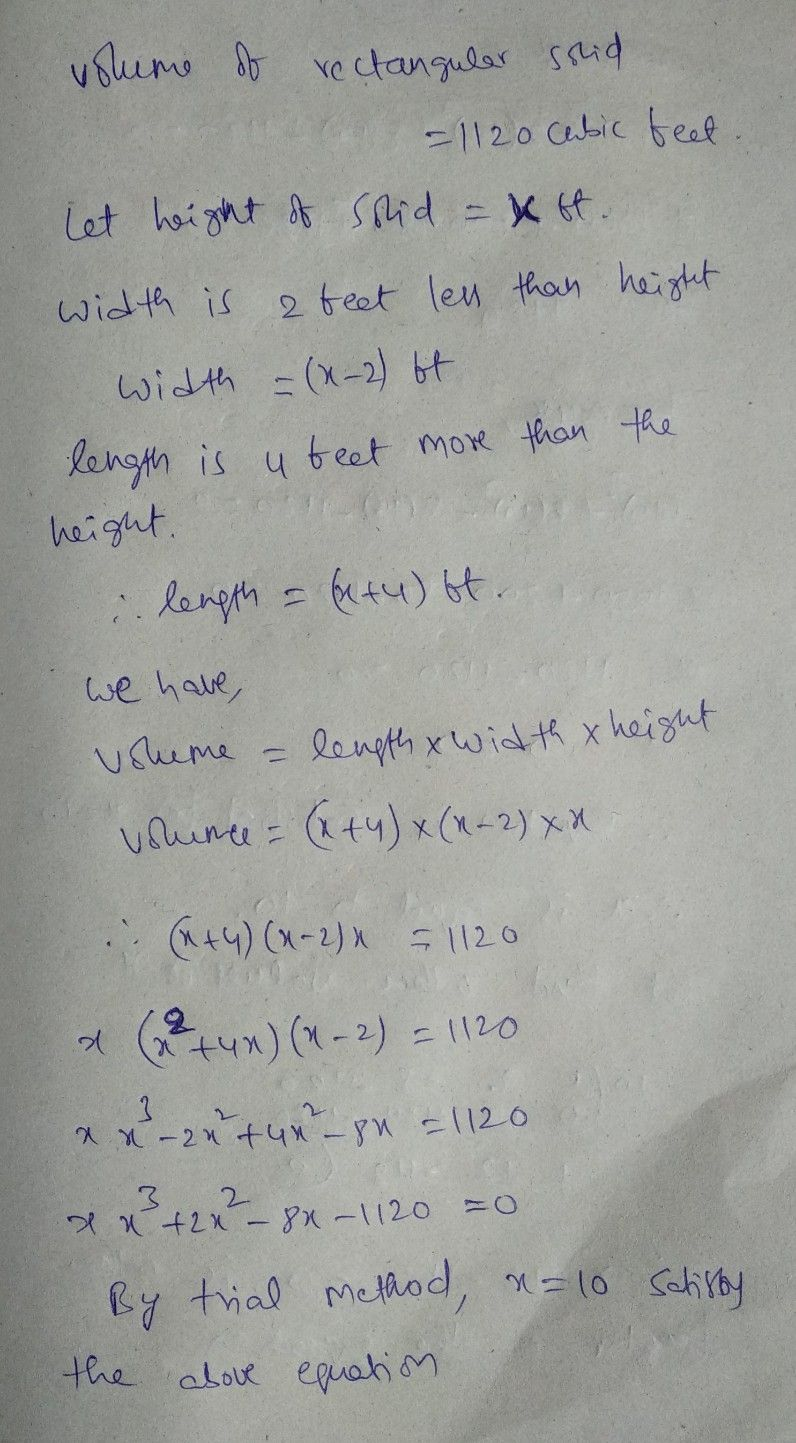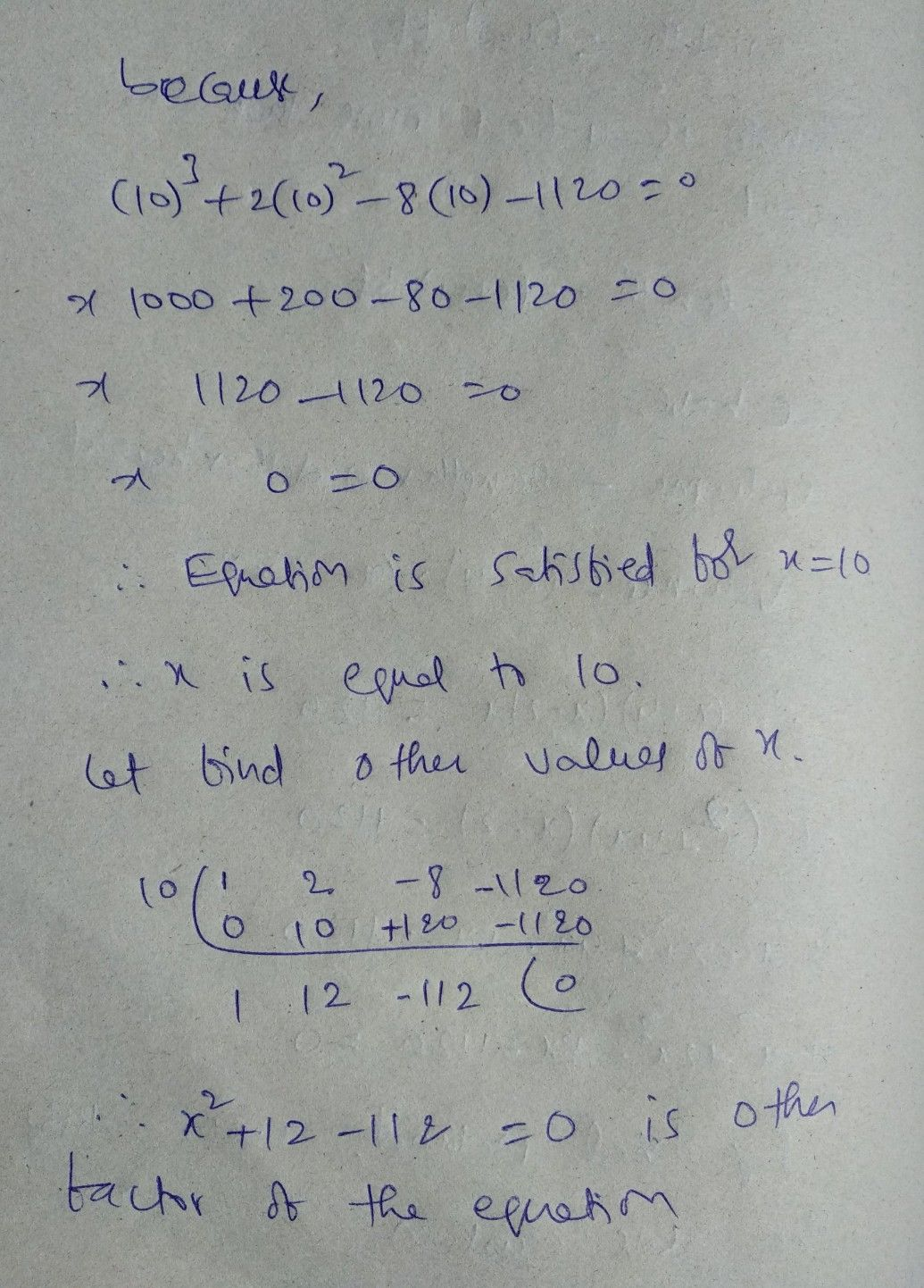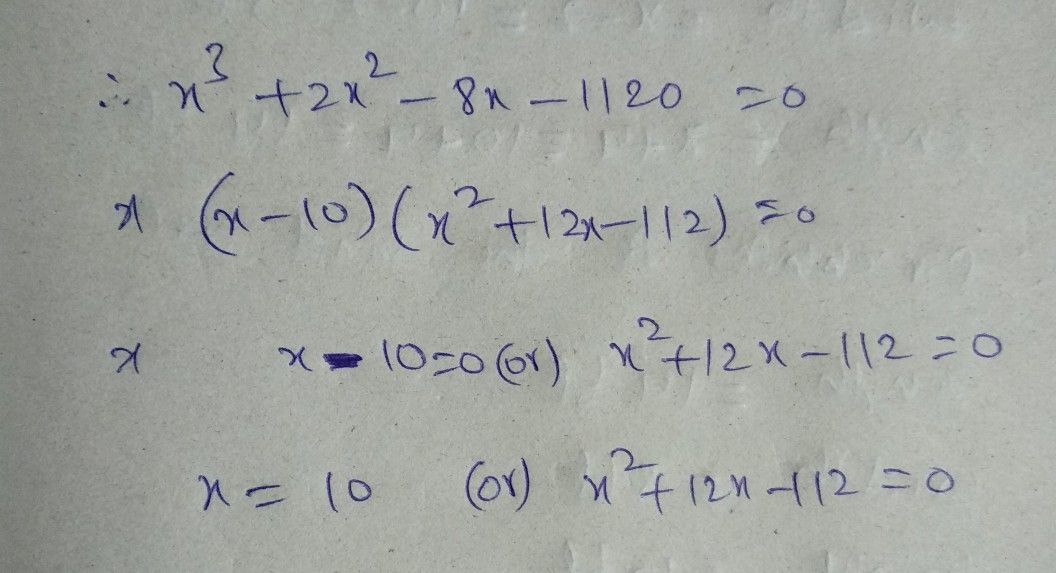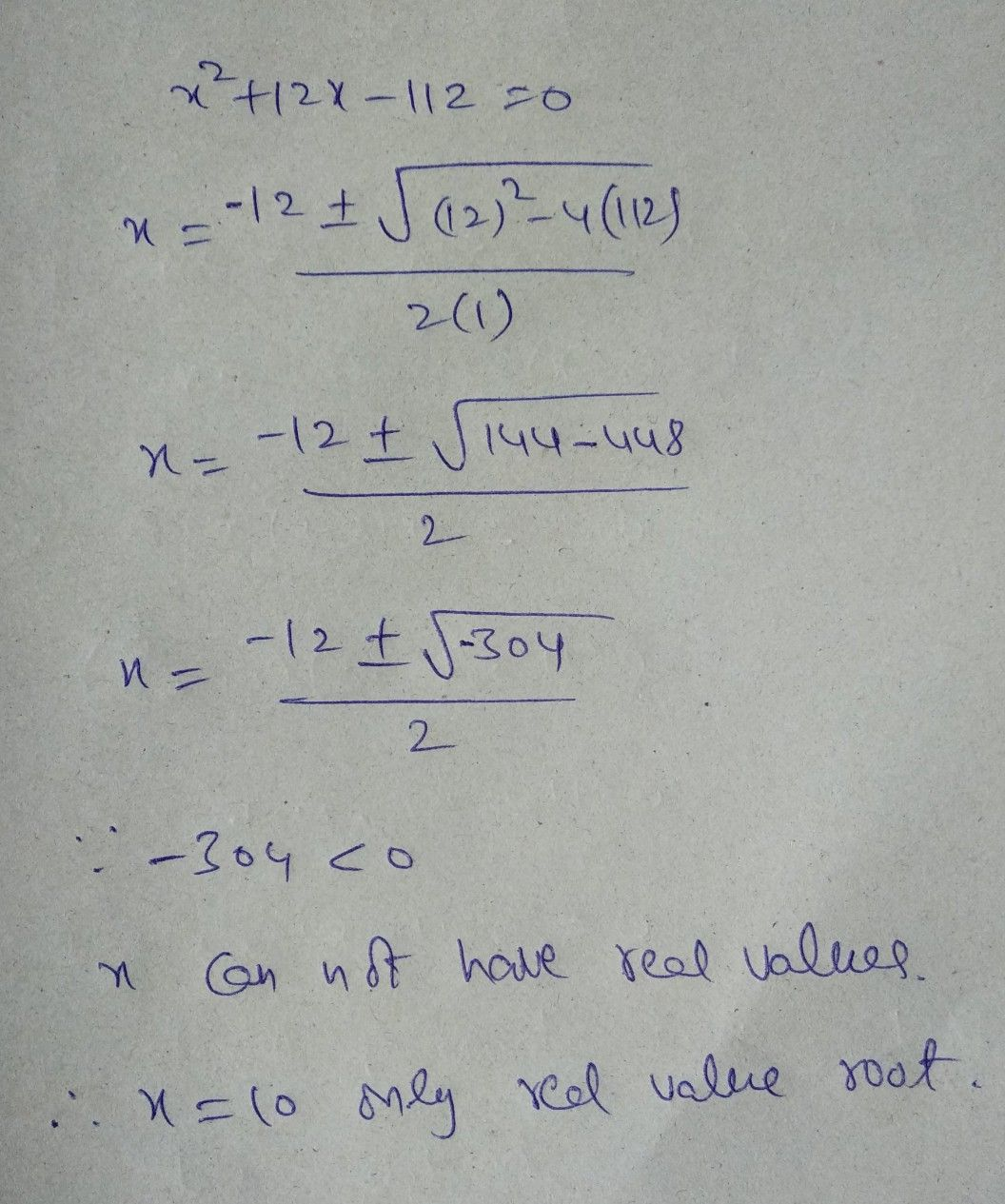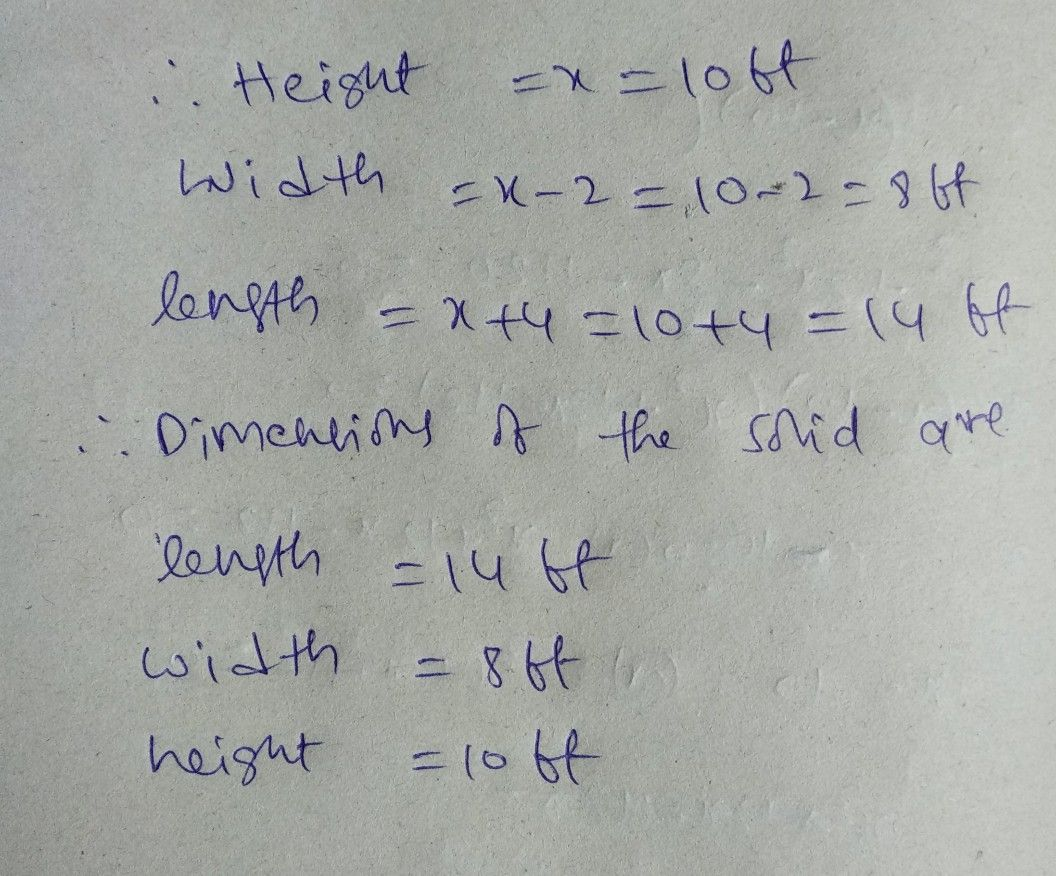Symbol
Problem$1.$ $GEOMETRY$ The volume of a rectangular solid is $1120$ cubic feet. The widthis $2$ feet less than the height, and the length is $41$ feet more than the height. Find the dimensions of the solid. $xft$ $\left(x-2\right)$ $H$ $\left(x-4\right)ft$